# How to Add a Unique ID Number Column for Duplicate Rows in Excel?

When we collect raw data in Excel, there is a chance that we will find duplicate data, which will affect or be redundant with our analysis. If the same data is present in Excel two or more times, then it can be called duplicate data.

When we collect the names of all the people in a group, there is a possibility that we have written a person's name twice. Adding a unique ID to each piece of data allows us to detect and remove duplicates.

In this tutorial, let us see a simple process to know how we can add a unique ID column for duplicate rows in Excel.

## Adding Unique ID Number Column for Duplicate Rows in Excel

In this case, we'll assign a number to the first element, then use the formula to get the next number, and finally use the autofill handle to finish the task. Let us see a simple process to know how we can add a unique ID column for duplicate rows in Excel.

Step 1

Let us open an Excel sheet where there will be a chance of duplication of the data, similar to the below image.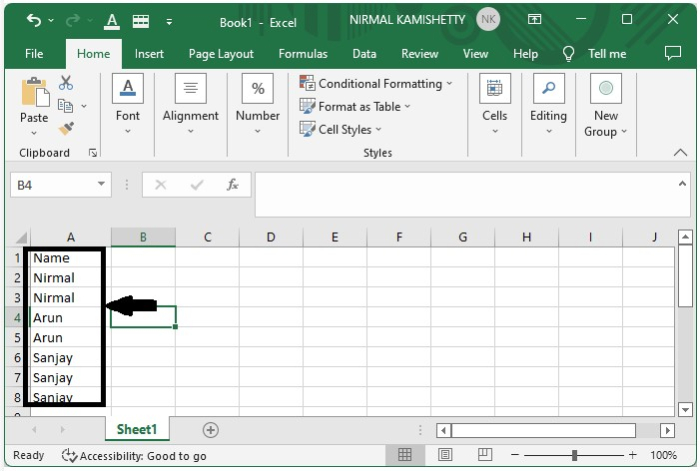As there are no empty cells to the left of the data to add the unique id, we need to move the data to the right side. We can do that by

Select data > Right click > Insert > Entire column > OK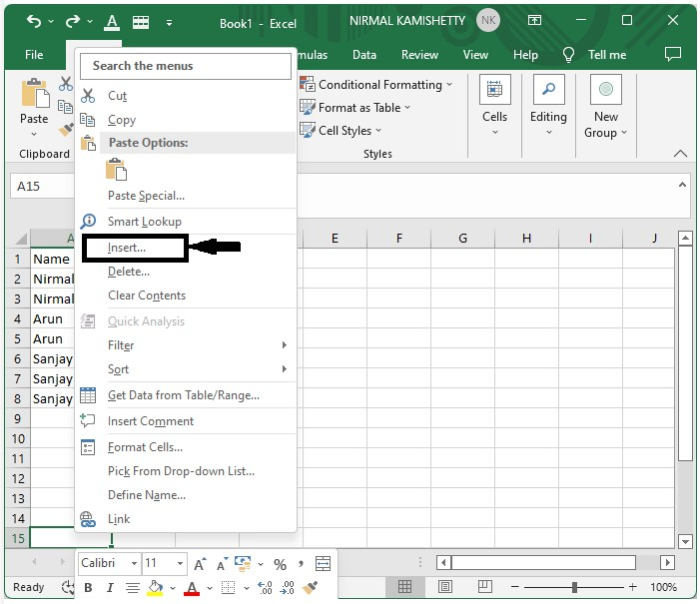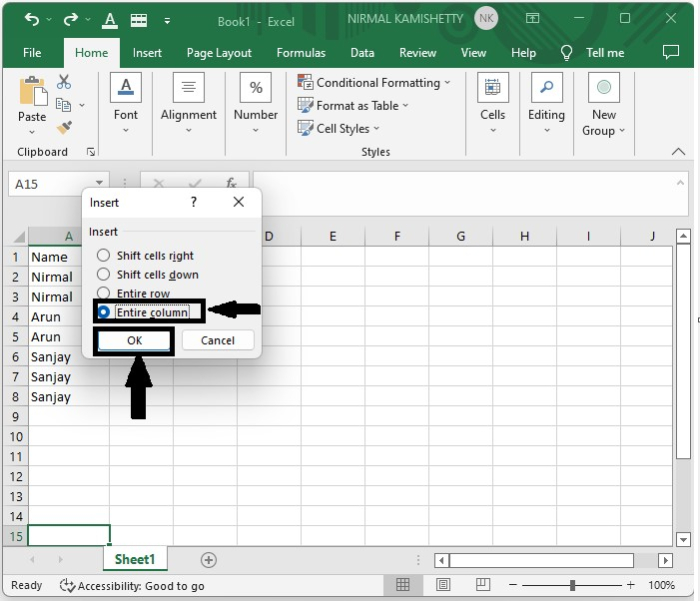Step 2

We successfully moved the entire row to the current time as a unique id in cell A1's address and set the first value to 1 in cell A2.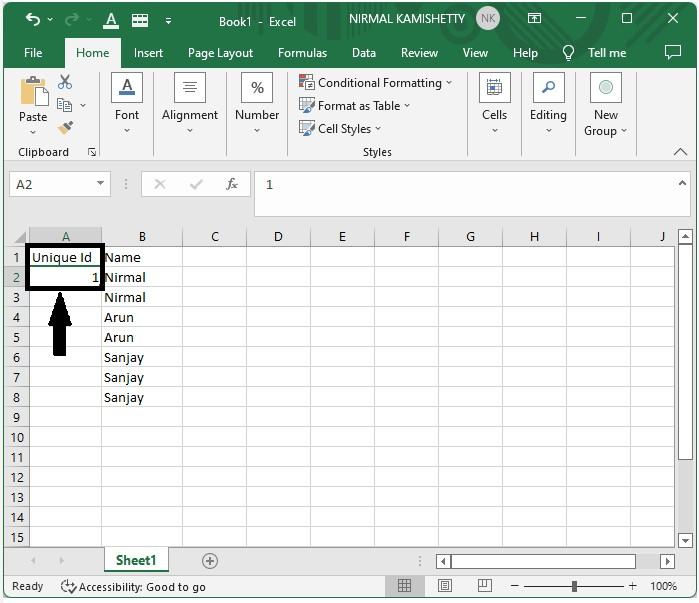Step 3

Now enter the formula as =IF(B2=B3, A2, A2+1) in the formula box in cell A3 to get the result for the second item, and click OK to get the result.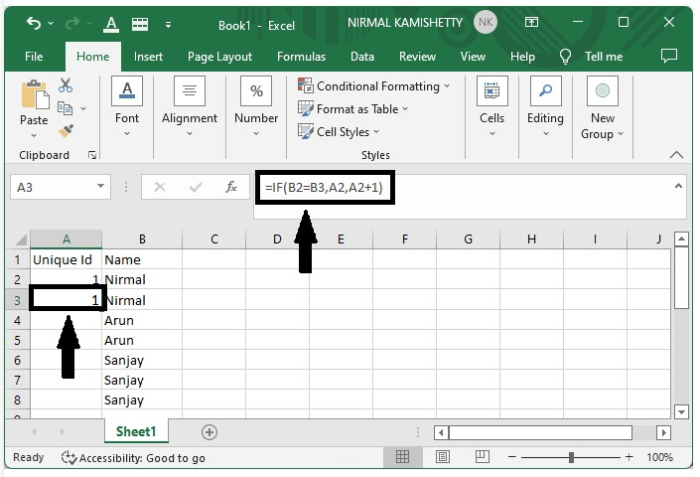Drag down from cell A3 using the auto-fill handle to get the final result shown in the image below.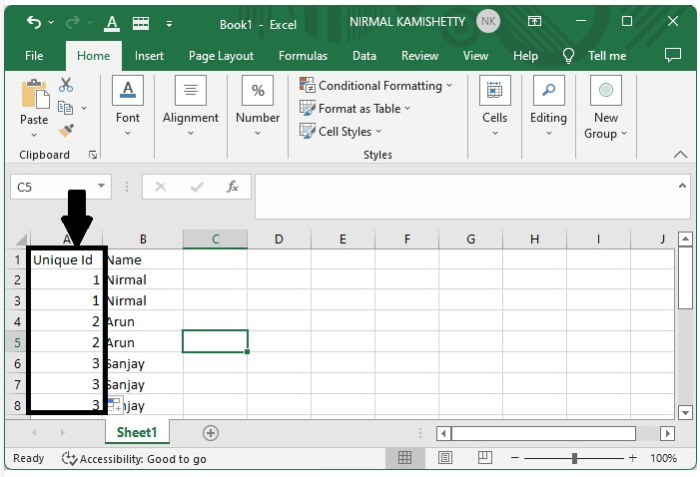## Conclusion

In this tutorial, we used a simple example to demonstrate how you can create a unique id for the number column for the duplicate rows in Excel to highlight a particular set of data.

Updated on: 09-Jan-2023

2K+ Views